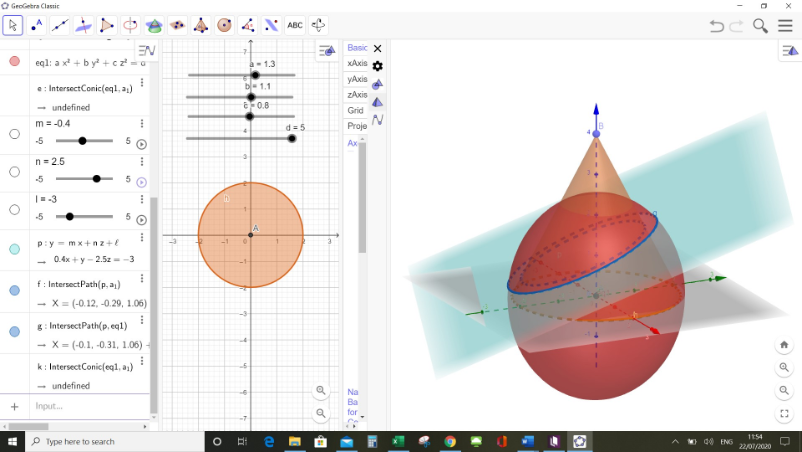# Overlapping Ellipse Game Challenge

## STEP-1: Making cone, ellipsoid and plane

1) Make a cone of radius = 2, height = 4 and initial point with coordinate (0,0,0). 2) Make an ellipsoid by entering following equation: ax^2 + by^2 + cz^2 = d. What shape did you get? 3) Now change sliders a,b,c and d to get an ellipsoid (refer to below image shown after step-1. 4) Enter equation of plane in input box: y=mx+nz+l 5) Once all three objects are appearing, change values of sliders m, n and l to get a plane which intersects both the cone and ellipsoid in such a way that an ellipse is visible to you where both plane and object intersects. You may need to change sliders a,b,c and d as well for achieving this.

## STEP-2: Defining intersection points

Enter the following in input box: 1) Intersect(name of cone, name of plane); For example (a,p) 2) Intersect(name of ellipsoid, name of plane). When you complete you should be getting a similar figure as shown below.Please note you may get something very dissimilar but only the requirements specified in step-1 are important; that your plane cuts the cone and ellipsoid in a manner that you get ellipse shaped intersections

## AND THE GAME STARTS!

Now try to move sliders such that we get two exactly same ellipses. 1) Did you manage to do so? 2) If yes, what can you conclude about ellipsoid and cone relationship? 3) If not, do you think it is not possible? Why?

## RIDDLE-2

Find intersection of cone and ellipsoid by using same method as shown in step 2 above. What did you get? Why did you get the result you got?

1) Now in the workspace above; you may hide the ellipsoid and now enter another equation: ﻿ ﻿ ﻿ ﻿ ﻿ ﻿ax^2 + by^2 - cz^2 = d 2) Find intersection of this object and the plane as in step-2 above. 3) Repeat the game now. What are your observations? Can you conclude anything?